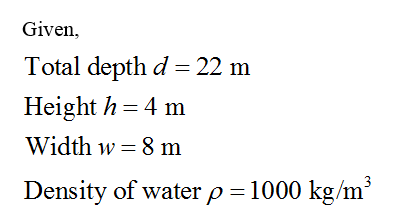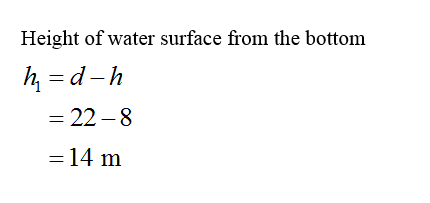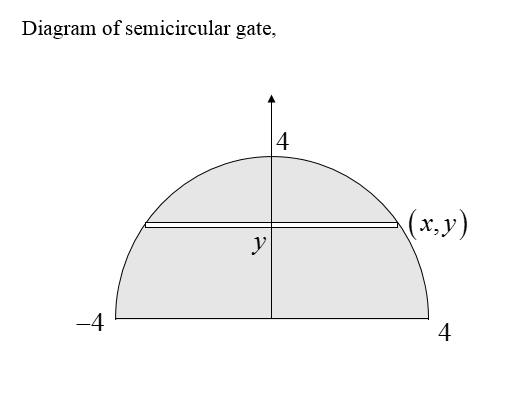Question
22 views

A vertical dam has a semicircular gate as shown in the figure. The total depth d of the figure is 22 m, the height h of air above the water level is 4 m, and the width w of the gate is 8 m. Find the hydrostatic force against the gate. (Round your answer to the nearest whole number. Use 9.8 m/s2 for the acceleration due to gravity. Recall that the weight density of water is 1000 kg/m3.)

check_circle

Step 1Step 2Step 3...

### Want to see the full answer?

See Solution

#### Want to see this answer and more?

Solutions are written by subject experts who are available 24/7. Questions are typically answered within 1 hour.*

See Solution
*Response times may vary by subject and question.
Tagged in